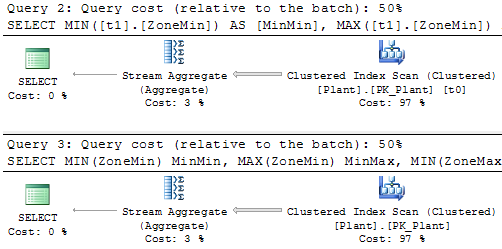## Friday, January 14, 2011

### Doing 2+ aggregations in LINQ to SQL

I was recently trying to do a simple double aggregation using LINQ to SQL.

Here's the setup:

One table "Plant" containing these two fields of concern:
`  ZoneMin tinyint  ZoneMax tinyint`

There's a search view on which I want to include the selection of a range for each of those fields. Therefore, I want to select the minimum and maximum of each field. In T-SQL this would be trivial:
`SELECT  MIN(ZoneMin) MinMin, MAX(ZoneMin) MinMax,  MIN(ZoneMax) MaxMin, MAX(ZoneMax) MaxMaxFROM Plant`

I tried numerous approaches with the .Aggregate() method, grouping and everything else I could think of. No luck. I finally broke down and posted to StackOverflow and got a simple answer. The end result is this LINQ query:
`Plants.GroupBy (p => 0).Select (p => new {  MinMin = p.Min (x => x.ZoneMin),  MinMax = p.Max (x => x.ZoneMin), MaxMin = p.Min (x => x.ZoneMax),  MaxMax = p.Max (x => x.ZoneMax)})`

This yields the following T-SQL (courtesy of LINQPad):
`-- Region ParametersDECLARE @p0 Int = 0-- EndRegionSELECT     MIN([t1].[ZoneMin]) AS [MinMin], MAX([t1].[ZoneMin]) AS [MinMax],     MIN([t1].[ZoneMax]) AS [MaxMin], MAX([t1].[ZoneMax]) AS [MaxMax]FROM (    SELECT @p0 AS [value], [t0].[ZoneMin], [t0].[ZoneMax]    FROM [Plant] AS [t0]    ) AS [t1]GROUP BY [t1].[value]`

Comparing the two execution plans (Query 2 is LINQ and Query 3 is T-SQL) shows that they are the same:So the lesson here is: remember to try constant value grouping.